< Prev
Next >

# Primitive Data Types and Sizes

In Java, each variable has a specific data type, where a data type tells us the size, range and the type of a value that can be stored in a variable. In Java, there are about eight primitive data types, such as - byte, short, int, long, char, float, double, boolean.

## Primitive data types are categorized into 4 parts

• integer data types, such as byte, short, int, long.
• floating-point data types, such as float, double.
• character data type, such as char.
• boolean data type, such as boolean.

Note: For many of us who like the reasoning behind equations, we will use a formula to calculate a minimum range and a maximum range of values, that can be stored for the data types like byte, char, int and long.

1. Minimum Range- It is the minimum value that can be stored in a data type.
2. Maximum Range- It is the maximum value that can be stored in a data type.

The minimum range is calculated by -2(bits-1) and for the maximum range, it is 2(bits-1)-1, while bits = size of a data type.

For example - The size of a byte data type is 8 bits. And, as per formula above, the minimum range of value that can be stored in a variable declared as the byte data type is -27(-128), while the maximum range of value is 27-1(127). Hence, the range of values for the byte data type is -128 to 127.

## Understanding primitive data types

Let's see each primitive data type in Java, one-by-one.

## byte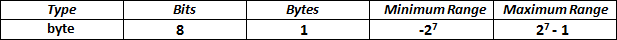• A variable of byte data type is of size 8 bits, which is equal to 1 byte.
• As per the formulas discussed above, a minimum value a byte variable can store is -27 or -128 and a maximum value in a byte variable is 27-1 or +127. Hence, it's range is -128 to 127.

• ## short• Size of a short variable is 16 bits or 2 bytes.
• A minimum value that can be stored in a short is -215 or -32768 and a maximum is 215-1 or +32767.
So, it's range is -32768 to 0 to 32767.

• ## int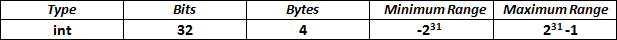• Size of an int variable is 32 bits or 4 bytes.
• Minimum value that can be stored in an int is -231 or -2, 147, 483, 648 while, Maximum is 231-1 or 2, 147, 483, 647. So, it's range is -2, 147, 483, 648 to +2, 147, 483, 647.

• ## long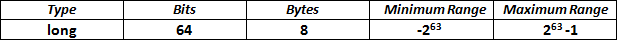• Size of a Java long variable is 64 bits or 8 bytes.
• The minimum range of a value that can be stored in a long is -263 while, the maximum is 263-1
Note: Because of a wider range than int, the long data type is used to store a larger number.

## float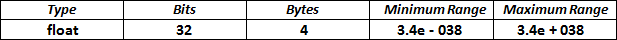• In float data types, we can hold a number with its fractional part as well. So, we use this data type when a very precise calculation is required.
• Size of a float variable is 32 bits= 4 bytes.
• You don't need to remember the minimum and maximum value floating data types but for those who still wish to know, its minimum range is 3.4e-038 and a maximum limit of 3.4e+038.

• ## double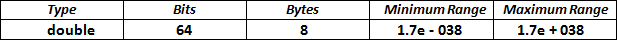• Size of a double variable is 64 bits= 8 bytes.
• Double data type variable may store a data with a minimum value of 1.7 e-038 until a maximum value of 1.7e+038

• Note: In double data type, we can hold numbers with double precision values, as compared to float.

## char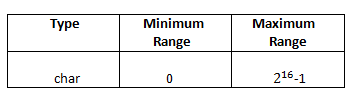• Java's char type variable takes 16 bits=2 bytes in the memory. In Java, we have Unicode characters. Unicode characters are represented by unsigned(only positive) 16-bit numbers. Hence, the minimum range is 0 and the maximum range is 216-1. Ranging from 0 to 65535 values.

• Why Unicode characters in Java?

• Because Unicode characters are 16 bits, a wider range than characters in C or C++(where they were just 8 bits or 1 byte). Hence in Java, a rich international set of characters are easily represented in Unicode.

## boolean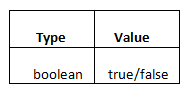• For boolean types, there is no range of values. Unlike programming languages like C/C++, a boolean primitive data type in Java cannot take a 0 or 1 value. A boolean in Java can be either true or false.

< Prev
Next >
< First Java Program
Class Access Modifiers >

Please subscribe to our social media channels for daily updates.